## Example Questions

### Example Question #1 : Geometry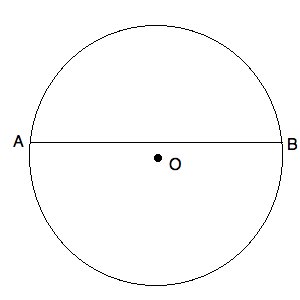O is the center of the circle above.

The length of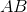is.

Quantity A: The area of the circle.

Quantity B: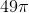Which of the following is true?

The relationship cannot be determined.

The two quantities are equal.

Quantity A is greater.

Quantity B is greater.

Quantity A is greater.

Explanation:O is the center of the circle above.

The length ofis.

Quantity A: The area of the circle.

Quantity B:Do not be tricked by this question. It is true thatcan be split into halves, each of which arein length. These halves are not, however, radii to the circle. Since this does not go through the center of the circle, its length is shorter than the diameter. This means that the radius of the circle must be greater than. Now, if it were, the area would be. Since it is larger than, the area must be larger than. Quantity A is larger than quantity B.

### Example Question #2 : Geometry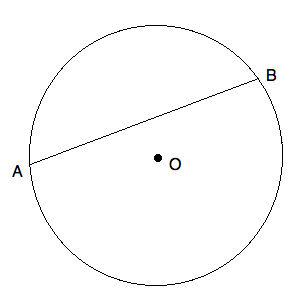O is the center of the circle above.

The circumference of the circle above is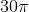.

Quantity A: The length of.

Quantity B:Which of the following is true?

Quantity A is larger.

The two quantities are equal.

The relationship cannot be determined.

Quantity B is larger.

Quantity B is larger.

Explanation:

Now, we know that the circumference of a circle is:or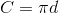This means that the diameter of our circle is must be. Given this, we know that themust be shorter than, for the diameter is the longer than any chord that does not pass through the center of the circle. Quantity B is larger than quantity A.

Tired of practice problems?

Try live online GRE prep today.